# Catch up on the latest AI articles# More Robust With Hostile Data Extensions + Mixups!

3 main points
✔️ Improve model robustness through adversarial data expansion and mixup
✔️ Establish a protocol for evaluating the robustness
✔️ Improve model robustness while maintaining model accuracy

written by Chenglei SiZhengyan ZhangFanchao QiZhiyuan LiuYasheng WangQun LiuMaosong Sun
(Submitted on 31 Dec 2020 (v1), last revised 6 Jun 2021 (this version, v3))

Subjects: Computation and Language (cs.CL)code：## Outline of Research

Known as BERT or RoBERTa, the Pretrained Language Models ( Pretrained Language Models), known as BERT and RoBERTa, are said to be vulnerable to Adversarial Attacks. For these pretrained language models, it is possible to A sentence in which some words of an input sentence are replaced by synonyms ( Adversarial Example) which substitutes some words of the input sentence with synonyms ("Adversarial Example") into the model, and Adversarial Attack has been discovered. To prevent such an attack and to make the To prevent such attacks and make the model more robust, there is Adversarial Training, which trains the model using Adversarial Examples.

However, the number of input sentences is infinite, and Adversarial Examples can be created to increase the training data, but the number of Adversarial Examples is insufficient and not exhaustive. Therefore, the authors also used a method called Mixup to linearly interpolate between the data and extend the training data.

## related research

We can expand the number of training data by creating Adversarial Examples for the training data. there are various methods to create Adversarial Examples, but in this paper, we use PWWS and TextFooler. (PWWS is a method that decides which words to replace in a sentence according to some evaluation function, and replaces words with synonyms one after another until the classification model fails to predict them.)

### Mixup Data Augmentation

Mix the two labeled data $(\mathbf{x}_i, \mathbf{y}_i)$ , $(\mathbf{x}_j, \mathbf{y}_j)$ and the beta distribution $\lambda\sim{Beta(\alpha,\alpha)}$ obtained from $\lambda \in [0 , 1]$ and mixes the data in the ratio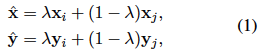Since input sentences (text) cannot be added together, in this paper, $\mathbf{x}_i,\mathbf{x}_j$ represent the output vectors of the {7,9,12}th layer of the model (BERT, RoBERTa).

We also name the method that mixes only [CLS] tokens asSMix and the method that mixes all tokens as TMix. In addition, $\mathbf{y}_i,\mathbf{y}_j$ is the One-Hot vector of labels for the classification task.

## proposed method

We used the Adversarial Data Augmentation and Mixup Data Augmentation mentioned in the related work to perform data augmentation and fine-tuning to improve the robustness of the model.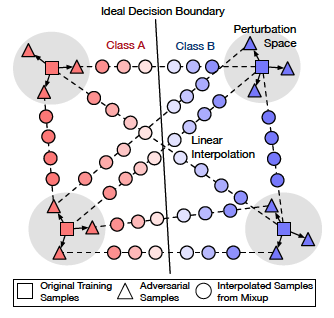As shown in this figure, there are three types of paired data for mixup: (original training data, original training data), (original training data, adversarial data), and (adversarial data, adversarial data).

Also, the loss function is the following equation.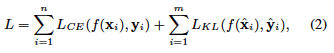$(\mathbf{x}_i, \mathbf{y}_i)$ represents the original training data and the created adversarial data, and the cross-entropy of the correct answer data $\mathbf{y}_i$ and the prediction $f(\mathbf{x}_i)$ is We calculate it. In addition, $(\hat{\mathbf{x}}_i, \hat{\mathbf{y}}_i)$ is the data created by mixup, and the KL of the correct answer label $\hat{\mathbf{y}}_i$ and the prediction $f(\hat{\mathbf{x}}_i)$ created by mixup is calculated. We calculate the divergence and sum it with the previous section to get the loss function.

## Evaluation Method

In this paper, we set up two evaluation methods to assess the robustness against Adversarial Attacks.

1. SAE (Static Attack Evaluation)
2. TAE (Targeted Attack Evaluation)

1. SAE: We create adversarial data for the original model (victim model) and use this created data to evaluate the accuracy against the new model.

2. TAE: After creating a model, we create adversarial data for that model as a victim model, and evaluate the accuracy of the victim model using this adversarial data. In other words, since we create adversarial data for each model and evaluate it with the model's own adversarial data, this is a more difficult evaluation method to improve the accuracy.

## Experiment & Analysis

### data set

In our experiments, we use the following three datasets.

1. Binary Classification: Emotional Analysis
1. SST-2
2. IMDB
2. 4-class classification: news topic classification
1. AGNews

### Experimental results 1

Now let's look at the results of the experiment. First, let's see how much the two evaluation methods (SAE and TAE) deviate from each other for a general fine-tuned model.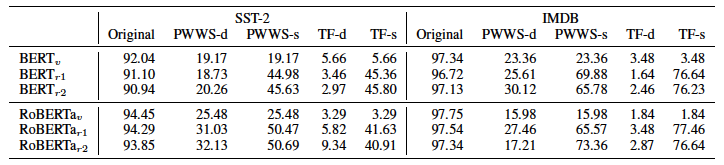BERT$v$ as the victim model and the fine-tuned model by changing only the random seed is BERT $r1$, BERT $r2$ and the same for RoBERTa. There are three test data used in the evaluation: the original test data, the data created by PWWS, and the data created by TextFooler (TF). (The original test data is used to create the data using PWWS and TF.)
For each data created by PWWS and TF, the results of accuracy by SAE (PWWS-s, TF-s) and accuracy by TAE (PWWS-d, TF-d) are included.

From this result, we can see that the accuracy in SAE is improved only by fine-tuning with changing only the random seed. On the other hand, it can be confirmed that the accuracy in TAE is low only by fine-tuning. Therefore, in the experimental result 2, we will see the result of the only TAE which is a more difficult evaluation method.

### Experimental results 2

Here are the results from SST-2 and IMDB. (AGNews is described later)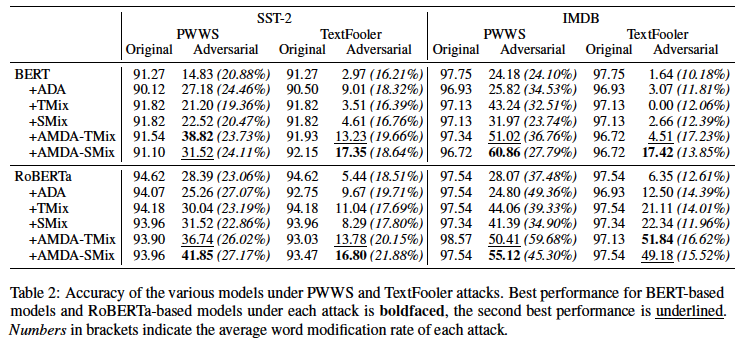First, we summarize the experimental setup. (For more details, please refer to Related Research and Proposed Methods.)

• TMix: mix all tokens during mixup
• SMix: Mix only [CLS] tokens during mixup
• AMDA-TMix: Using TMix in AMDA, the proposed method
• AMDA-SMix: Using SMix in AMDA, the proposed method

The results of RoBERTa show that the robustness is improved even when only Mixup (TMix, SMix) is used. The robustness of the proposed method is improved from the baseline in both tasks, suggesting that Adversarial Data Expansion (ADA) and Mixup complement each other and contribute to the robustness improvement.

The results also show that the accuracy of the original test data is reduced when using adversarial data augmentation (ADA), but the proposed method is compared to Adversarial Data Augmentation (ADA). Some of the results show that the accuracy against the original test data is also improved.

The number in parentheses in the table of results is the average ratio of word substitutions per data when creating adversarial data. In the proposed method, this higher ratio can be interpreted to mean that the creation of adversarial data is more difficult, and the robustness of the proposed method is improved.

Below are the results from AGNews.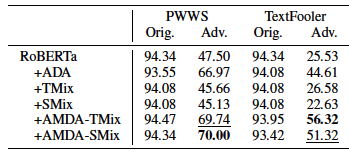The results here are similar to those in SST-2 and IMDB.

## summary

In this paper, we proposed a fine-tuning method using adversarial data extension and Mixup. We also set up two evaluation methods to check the robustness against adversarial data. We evaluated the proposed method using TAE, which is the most difficult evaluation method among them and showed the robustness of the proposed method.
In particular, the setting of the evaluation method in this paper is a useful indicator for those who do similar research to compete for accuracy.

If you have any suggestions for improvement of the content of the article,